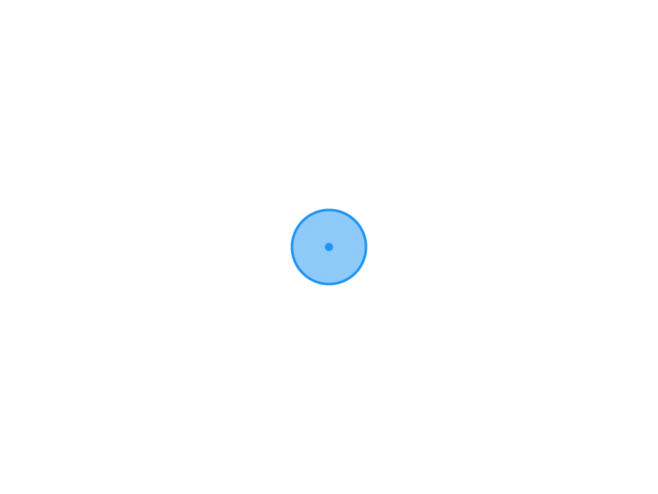TypechoJoeTheme

# 日志随记

/XG.孤梦

# C语言字符串与指针-学习二十五2022-02-20
/
0 评论
/
729 阅读
/
932 个字
/

02/20

## 字符串的引用方式

• 字符串是存放在字符数组中的。
• 引用一个字符串,可以用以下两种方法：

• 字符数组存放一个字符串，可以通过数组名和格式声明 “%s” 输出该字符串也可以通过数组名和下标引用字符串中一个字符。
• 字符指针变量指向一个字符串常量，通过字符指针变量引用字符串常量
• 例子
``````#include <stdio.h>
void main() {
char string[] = "I love China!";
printf("%s\n", string);
printf("%c\n", string); // 输出第八个字符
}

//通过字符指针输出一个字符串

#include <stdio.h>
void main() {
char *string = "I love China!";
printf("%s\n", string);
printf("%c\n", string); // 输出第八个字符
}````````````#include <stdio.h>
void main() {
char a[] = "abcdefg";
char b;
int i;
for (i = 0; *(a + i) != '\0'; i++) {
*(b + i) = *(a + i);
}
*(b + i) = '\0';
printf("a = %s\n", a);
// printf("b = %s\n", b);
printf("b = ");
for (i = 0; b[i] != '\0'; i++) {
printf("%c", b[i]);
}
printf("\n");
}````````````#include <stdio.h>
void main() {
char a[] = "abcdefg", b,*p1,*p2;
p1 = a;
p2 = b;
for (; *p1 != '\0'; p1++) {
*p2 = *p1;
p2++;
}
*p2 = '\0';
printf("a = %s\n", a);
printf("b = %s\n", b);
}``````## 字符指针变量和字符数组的比较

• 字符数组字符指针变量都能实现字符串的存储和运算
它们二者之间是有区别的，主要有以下几点:

• 1.字符数组若干个元素组成，每个元素中放一个字符
字符指针变量中存放的是地址（字符串第1个字符的地址），而不是将字符串放到字符指针变量中。
• 2.赋值方式不同，可以对字符指针变量赋值,但不能对数组名赋值

• `char *a;` `a="I love Chinal";` 正确
• `char str;` `str='1';` 正确
• char str; str = "I love China!"; 错误
• 3.存储单元的内容不同，编译时为字符数组分配若干存储单元以存放各元素的值,
而对字符指针变量，只分配一个存储单元

• `char *a, str;`
`a=str;` `scanf ("%s",a);` 正确
• char *a; scanf("%s",a); 错误
• 4.指针变量的值是可以改变的，而数组名代表一个固定的值（即数组首元素的地址）不能改变
• 例子
``````#include <stdio.h>
void main() {
char *a = "I love China!";
a = a + 7;
printf("%s\n", a); // 从第八个字符开始输出
}``````• 5.字符数组各元素的值是可以改变的,但字符指针变量指向的字符串常量中的内容是不可以被取代的。

• `char a[]="House", *b="House";`
• `a='r';` 正确
• b='r'; 错误
• 6.引用数组元数

• 对字符数组可以用下标法地址法引用数组元素。

• 例如：`a``*(a+5)`
• 如果字符指针变量`p=a`，则也可以用指针变量带下标的形式和地址法引用。

• 例如：`p``*(p+5)`
• 7.用指针变量指向一个格式字符串,可以用它代替printf函数中的格式字符串
• 例子
``````#include <stdio.h>
void main() {
char *format;
int a = 10;
float b = 3.6;
format = "a = %d, b = %f \n";
printf(format, a, b);
// 相当于
printf("a = %d, b = %f \n", a, b);
}``````## 字符指针作函数参数

• 如果想把一个字符串一个函数“传递”到另一个函数，可以用地址传递的办法,
即用字符数组名参数,也可以用字符指针变量参数
• 被调用的函数中可以改变字符串的内容，在主调函数中可以引用改变后的字符串
• 例子

``````#include <stdio.h>

void main(){
// 字符数组名做参数
void string_copy(char from[], char to[]);
char a[] = "abcdefg", b;
string_copy(a, b);
printf("a = %s\n", a);
printf("b = %s\n", b);
}

void string_copy(char from[], char to[]){
int i;
for (i=0; from[i]!= '\0'; i++) {
to[i] = from[i];
}
to[i] = '\0';
}``````
``````#include <stdio.h>

// 字符指针变量做参数
void main(){
void string_copy(char *from, char *to);
char *a = "abcdefg", b;
char *to = b;
string_copy(a, to);
printf("a = %s\n", a);
printf("b = %s\n", b);
}

void string_copy(char *from, char *to){
for (; *from != '\0'; from++,to++) {
*to = *from;
}
*to = '\0';
}``````## 指向函数的指针

• 在C语言中,一个函数总是占用一段连续的内存区，而函数名就是该函数所占内存区的首地址
• 我们可以给这个首地址(或称入口地址)赋予一个指针变量,使该指针变量指向该函数
• 然后通过指针变量就可以找到并调用这个函数。我们把这数的指针变量称为“函数指针变量”

### 用函数指针变量调用函数

• 函数指针变量的定义

• 一般形式为:

• `类型说明符` `(*指针变量名)()`
• 类型说明符：表示被指函数的返回值的类型
• *指针变量名：“*”后面的变量是定义的指针变量
• ()：指针变量所指的是一个函数
• 例如：`int (*pf)();`
表示 pf 是一个指向函数入口的指针变量，(函数值)是整型。
• 例子
``````#include <stdio.h>

// 字符指针变量做参数
void main(){
int max(int, int);
int(*p)(int, int);
int a, b, c;
p = max;
scanf_s("%d %d", &a, &b);
c = (*p)(a, b);
printf("max=%d\n", c);
}

int max(int x,int y){
int z;
z = (x > y) ? x : y;
return (z);
}``````https://www.xggm.top/archives/664.html（转载时请注明本文出处及文章链接）XG.孤梦博 主大佬

51 文章数
106 评论量

X